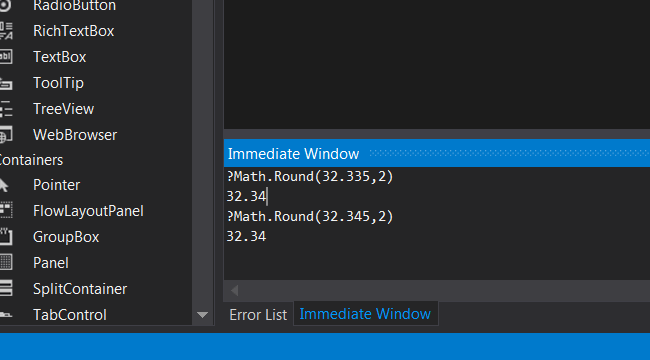#### What'stheproblemwithroundingnumbersin.NET?I have been involved lately in a soon-to-be-huge project about accounting, invoices, etc.

When printing some invoices I noticed some inconsistencies in the sums; one or two cents difference with respect to the result I obtain when checking the numbers by hand. A little digging and the problem was isolated; it was the Math.Round() function.

##### Replicating the problem

At the immediate window (press Ctrl+G to open it in the editor), type:

The result is:

Then type:

###### ?Math.Round(32.345,2)

The result is the same:

##### Solution

My first impression was "oh my, Microsoft reaaaaaaally messed up this time!".

But no: Math.Round() uses Banker's rounding by default. From MSDN:

Rounding away from zero

Midpoint values are rounded to the next number away from zero. For example, 3.75 rounds to 3.8, 3.85 rounds to 3.9, -3.75 rounds to -3.8, and -3.85 rounds to -3.9. This form of rounding is represented by the MidpointRounding.AwayFromZero enumeration member. Rounding away from zero is the most widely known form of rounding.

Rounding to nearest, or banker's rounding

Midpoint values are rounded to the nearest even number. For example, both 3.75 and 3.85 round to 3.8, and both -3.75 and -3.85 round to -3.8. This form of rounding is represented by the MidpointRounding.ToEven enumeration member. Rounding to nearest is the standard form of rounding used in financial and statistical operations. It conforms to IEEE Standard 754, section 4. When used in multiple rounding operations, it reduces the rounding error that is caused by consistently rounding midpoint values in a single direction. In some cases, this rounding error can be significant.

##### Conclusion

There you have it. You need to use Math.Round(value, digits, MidpointRounding.AwayFromZero) to have the desired effect (i.e. the "normal" rounding). I have my reservations about using the banker's rounding as default, since it is acknowledged that rounding away from zero is the most widely known form of rounding.